V.5 No 2 27 On light aberrationSpeaking of possibility of non-interferometric measurements of the speed of absolute motion of observer free of considerable masking effects, which are inherent in interferometric methods, let us additionally consider the following conceptual experimental method. Truly speaking, it has no direct concern to the aberration effect. This method is based on the measurement of phases of emission of two frequencies emitted by the common emitter of microwave range. These frequencies pass simultaneously the common distance from the emitter 3 and receiver 4. To make it practically, we have to set the emitter and receiver onto some rigid underpinning able to turn by 360oaround its axis, as shown in Fig. 11.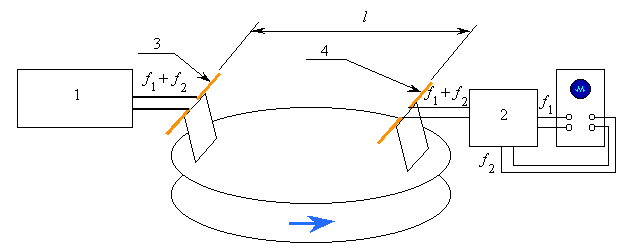Fig. 11. The scheme of the method to measure the phase difference: 1 is the generator, 2 is the receiver, 3 is the emitting antenna, and 4 is the receiving antenna
 To describe this method mathematically, suppose, the emitter simultaneously emits two strongly monochromatic EM waves towards the receiver. The E-components of these waves we can write as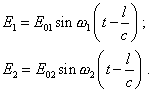(72)
 These waves1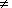2 are simultaneously registered by the receiver, selected and put to two inputs of two-ray oscillograph or to the x- and y-plates of one-ray oscillograph, dependently on the method to register the phase difference. We see from (72) that the current time t is common for both rays. The frequencies1 and2 also remain constant from the view of both formalisms, as the source and receiver are stationary as to each other. The phase of received signal is in fact determined by the terms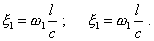(73)
 Thus,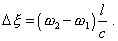(74)
 The further description of the method we will conveniently give, noting the particular features of classical and relativistic formalisms.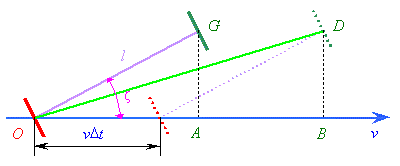Fig. 12. The graph for calculation of delay of EM wave propagating along the moving measuring rod from the view of classical formalism
 From the view of classical formalism, the calculation can be made on the basis of Fig. 12. From the graph,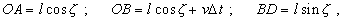(75)
 where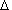t is the time necessary the ray to pass from the source to receiver, hence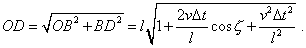(76)
 At small velocities of the frame, we can approximately write (76) as follows: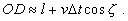(77)
 Noting that from the view of classical formalism the EM wave propagation speed is constant in relation to the aether, we can write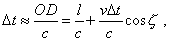(78)
 or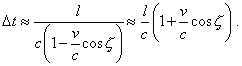(79)
 Multiplying the right and left parts of (79) accordingly by1 and2 , yield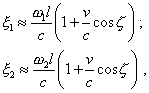(80)
 and from (80) yield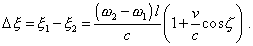(81)
 We see from (81) that the sought effect of the phase of two received signals depends on the first order of ratio v/c and can be regulated, if we change the difference of frequencies received by the receiver, and can be less changed by the size of base on which the emitter and receiver are fixed. To write mathematically the effect expected from the view of relativistic formalism, we can use the conclusions of basic relativistic theory, which states that the speed of light in the accompanying frame is strongly constant and the observer is unable to independently measure the difference in longitudinal and transverse sizes. With this approach, we will see no changes when the device turns. Thus, the described method allows to reveal and to experimentally study the effect of frame’s motion in relation to the aether. From the view of classical formalism, the phase difference has to change with the turn of device, describing some cardioid, as shown in Fig. 13. And this change of phase is the effect of first order of the ratio v/c, which, after relativistic opinion, cannot be realised in the experiments of such kind.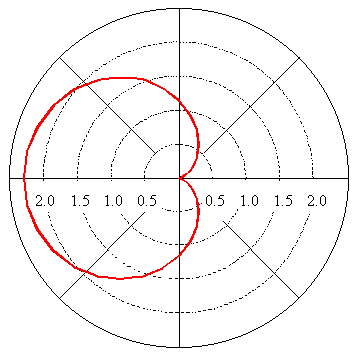Fig. 13. The change of phase difference of received two-frequency signal plotted against the angle of device turn to the aethereal wind, according to classical formalism